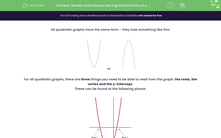# Identify and Interpret the Significant Points of a Quadratic Graph

In this worksheet, students will practise identifying the roots, vertices and intercepts of a quadratic graph.Key stage:  KS 4

Year:  GCSE

GCSE Subjects:   Maths

GCSE Boards:   AQA, Eduqas, OCR, Pearson Edexcel,

Curriculum topic:   Algebra, Graphs of Equations and Functions

Curriculum subtopic:   Graphs Graphs of Equations and Functions

Difficulty level:#### Worksheet Overview

All quadratic graphs have the same form - they look something like this:For all quadratic graphs, there are three things you need to be able to read from the graph: the roots, the vertex and the y-intercept.

These can be found at the following places:The roots occur where the graph crosses (or touches) the x-axis. You need to remember that for a quadratic, there could be either two roots(as in the example), one root (if the graph just touches the x-axis) or even zero roots (if the graph is above the x-axis).

The intercept occurs where the graph crosses the y–axis.

The vertex, also called the turning point, is the lowest (or highest if the quadratic is negative) value of the graph.

Example:

Find the significant points on this quadratic graph:This is just a case of reading them off the graph.

The roots are at x = -3 and x = 4

The intercept is at y = -12

The vertex is a little harder to read as it isn’t exactly on a point. When you get this situation, remember that you will get some leeway.

This point also has to be given as a coordinate as it is not on an axis. (0.5, -12.2)

Time for some questions now.

### What is EdPlace?

We're your National Curriculum aligned online education content provider helping each child succeed in English, maths and science from year 1 to GCSE. With an EdPlace account you’ll be able to track and measure progress, helping each child achieve their best. We build confidence and attainment by personalising each child’s learning at a level that suits them.

Get started